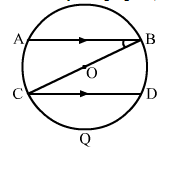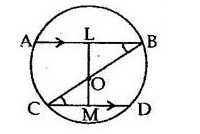# In the adjoining figure, BC is a diameter of a circle with centre O.

Question:

In the adjoining figure, BC is a diameter of a circle with centre O. If AB and CD are two chords such that AB || CD, prove that AB = CD.Solution:

Given: BC is a diameter of a circle with centre O. AB and CD are two chords such that AB || CD.
TO prove: AB = CD
Construction: Draw OL  AB and OM  CD.Proof:
In ΔOLB and ΔOMC, we have:
∠OLB = ∠OMC        [90° each]
∠OBL = ∠OCD          [Alternate angles as AB || CD]
OB = OC                      [Radii of a circle]
∴ ΔOLB ≅ ΔOMC   (AAS criterion)
Thus, OL = OM   (CPCT)
We know that chords equidistant from the centre are equal.
Hence, AB = CD